Скачать презентацию 12 Power Distribution Systems 1 Schematic diagram of

c53b7cee984043ebab64042a5ed95d8a.ppt

• Количество слайдов: 9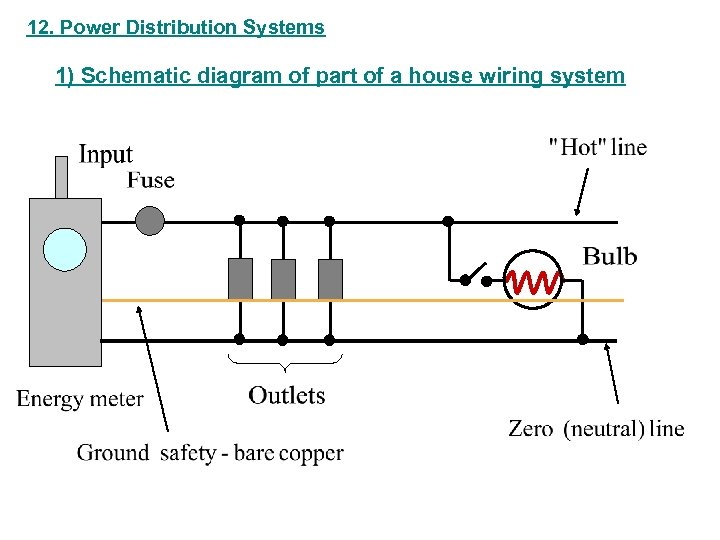12. Power Distribution Systems 1) Schematic diagram of part of a house wiring system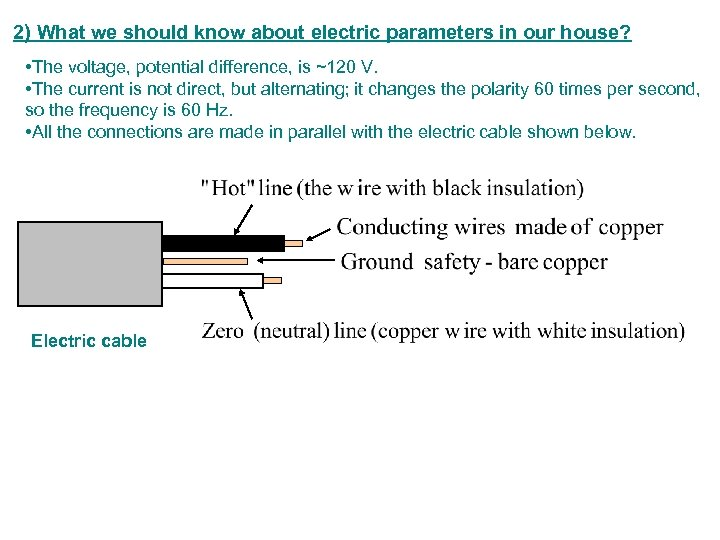2) What we should know about electric parameters in our house? • The voltage, potential difference, is ~120 V. • The current is not direct, but alternating; it changes the polarity 60 times per second, so the frequency is 60 Hz. • All the connections are made in parallel with the electric cable shown below. Electric cable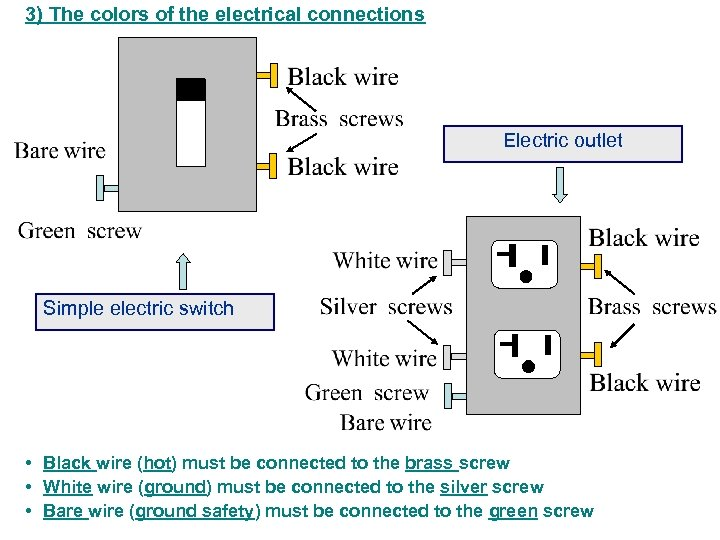3) The colors of the electrical connections Electric outlet Simple electric switch • Black wire (hot) must be connected to the brass screw • White wire (ground) must be connected to the silver screw • Bare wire (ground safety) must be connected to the green screw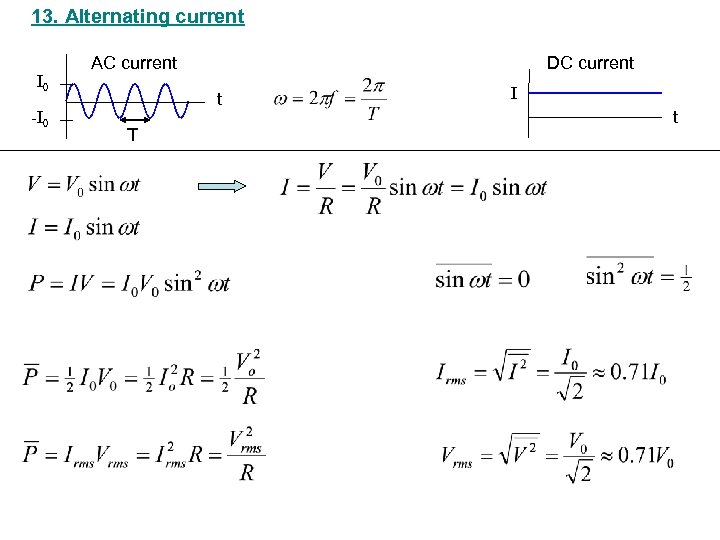13. Alternating current I 0 -I 0 AC current DC current t T I t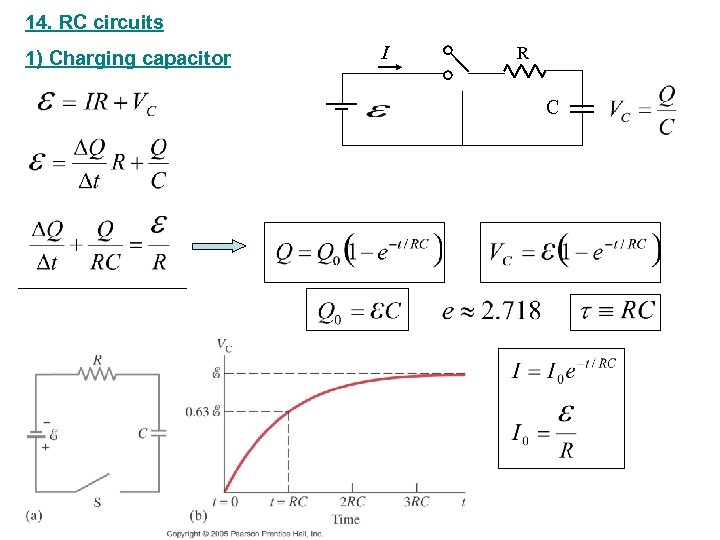14. RC circuits 1) Charging capacitor I R C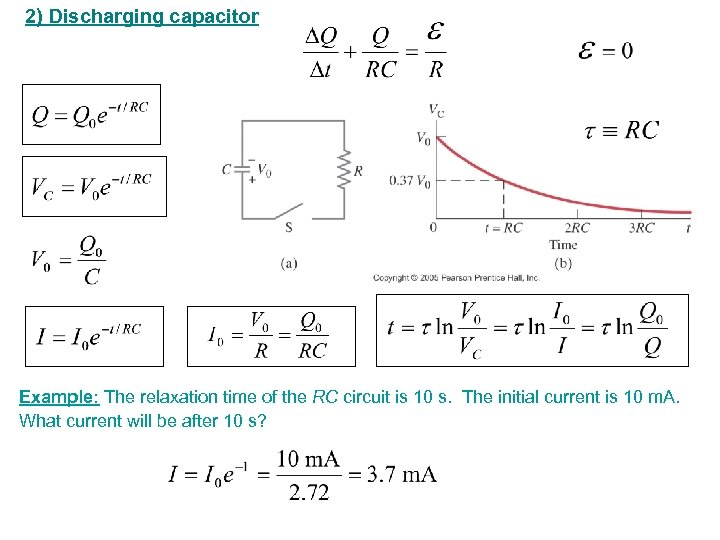2) Discharging capacitor Example: The relaxation time of the RC circuit is 10 s. The initial current is 10 m. A. What current will be after 10 s?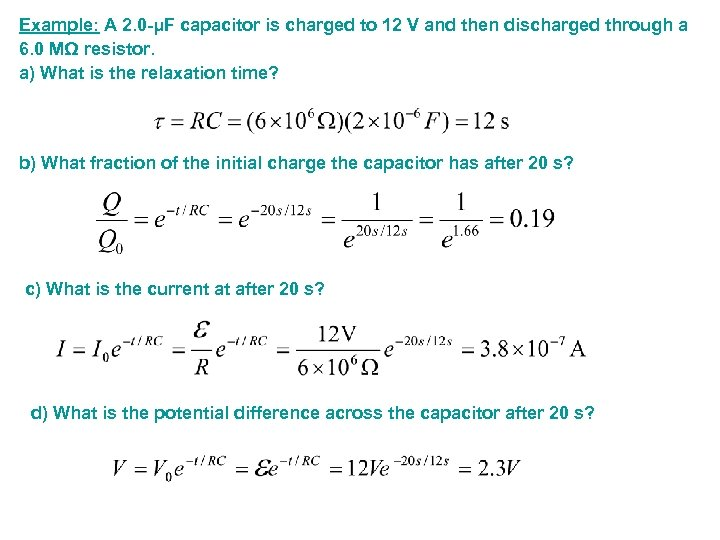Example: A 2. 0 -μF capacitor is charged to 12 V and then discharged through a 6. 0 MΩ resistor. a) What is the relaxation time? b) What fraction of the initial charge the capacitor has after 20 s? c) What is the current at after 20 s? d) What is the potential difference across the capacitor after 20 s?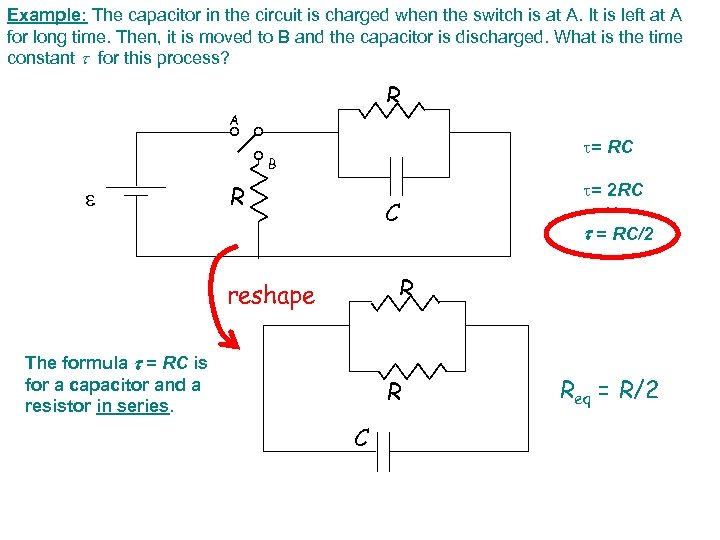Example: The capacitor in the circuit is charged when the switch is at A. It is left at A for long time. Then, it is moved to B and the capacitor is discharged. What is the time constant for this process? R A t= RC B R C t= 2 RC = RC/2 R reshape The formula = RC is for a capacitor and a resistor in series. R C Req = R/2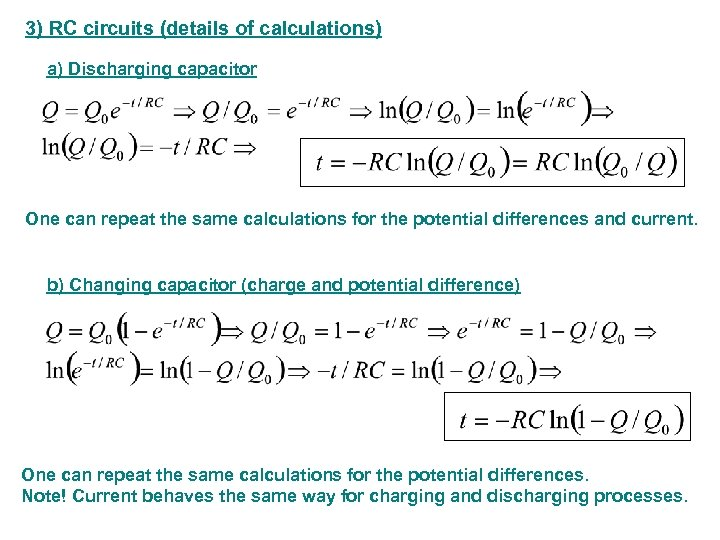3) RC circuits (details of calculations) a) Discharging capacitor One can repeat the same calculations for the potential differences and current. b) Changing capacitor (charge and potential difference) One can repeat the same calculations for the potential differences. Note! Current behaves the same way for charging and discharging processes.